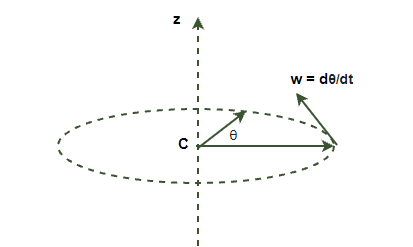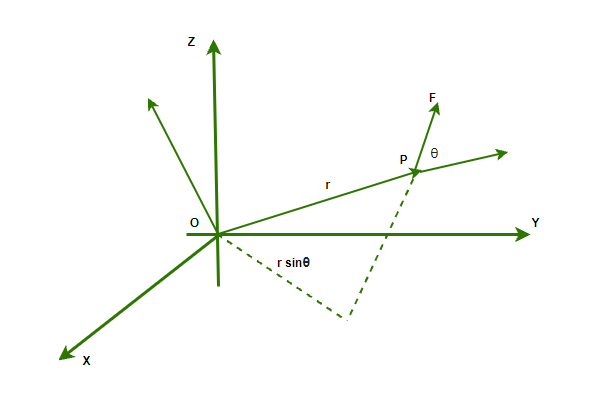Open In App
Related Articles
• CBSE Class 11 Physics Notes

# Rigid Body – Definition, Rotation, Angular Velocity, Momentum

Rigid body rotation is featured prominently in science, sports, and engineering. Theoretically, it is a collection of particles that are at a fixed distance from one another. In an ideal scenario, these bodies do not change their shape or deform. These kinds of bodies are usually a continuous distribution of mass. For analyzing the motion of such bodies, the position of the center of mass is required. When forces are applied to such bodies, it produces an angular acceleration and linear acceleration. It becomes essential to study how these forces affect rigid bodies and produce an angular and linear acceleration in them. Let’s see them in the detail.

### Rigid Body Rotation

Rigid body rotation is a motion that occurs when a solid body moves in a circular path around something. The rotational motion can be broken down into two types of rotation – Rotation about a fixed axis and rotation about a fixed point. Rotation about a fixed axis is said to be when the body is rotating about an axis that has a fixed location and orientation relative to the body. Example of such rotations includes – hinged door. The second type of rotational motion involves the rotation of the body around a point. A child’s spinning top is one example of this type of rotational motion.

### Angular Velocity

The angular velocity is defined as the angular displacement with respect to time. For example, a motorcycle going around on a circular track in the well of death, a merry-go-round, etc. For a body that is rotating around some fixed axis, the angle covered by the body changes with time. Let’s denote the angle covered by θ, the angular velocity is defined as the rate of change of the angle of the body. It is denoted by ω. For the body which is rotating at a constant rate, the average angular velocity can be used,In cases where the rotational motion is not constant, instantaneous angular velocity is calculated.

ω = dθ/dt

### Angular Momentum

Force is required to bring the body into translatory motion. There are parallels between the rotation and translatory motion. Many quantities such as displacement, acceleration, velocity, etc have corresponding values in the rotational domain. Torque is analogous to force in rotational motion. Torque brings a change in the angular momentum of the system. Angular momentum is analogous to linear momentum in the case of translatory motion. In the figure, a particle is given whose position vector with respect to the origin O is “r”. “p” denotes the linear momentum of the particle moving around that point. In that case, the angular momentum is given by,

l = r × p

This is the crossproduct of the position vector and the linear momentum vector. The magnitude of the vector is given by,

l = rpsin(θ)The relation between the torque and force can also be derived from these equations. Differentiating the above equation,

l = r × pτ = r × F

In the case of angular momentum of a system of particles, the angular momentum of the system is given by the vector sum of the individual momentum of the particles.

L = l1 + l2 + l3 + .. ln

L = r1 × p1 + r2 × p2 + r3 × p3 …. rn × pn

### Torque

Torque is analogous to the force in the case of rotational motion. This is also called the moment of force. This causes angular acceleration in the body, which means forcing to change the rotational state of the body. Imagine opening or closing the door of a room, in this case, the motion of the door is circular motion, and therefore, torque is applied on the door. In short, the force applied to move a body in circular motion is torque. Let a particle P be located far from the origin at a point whose position vector is given by, If the force F is acting on the particle, the moment of force is given by,It is a vector quantity. The magnitude of the torque is given by,

τ = F.r.sinθ

Here, “r” is the magnitude of the position vector, and θ is the angle between the position vector and force. In the formula, the part “rsinθ” is the perpendicular distance of the force from the axis of the rigid body.

Dimensions of torque are given by ML2T-2. These are also dimensions of energy but, these quantities should not be confused as similar. They are very different quantities.The unit of torque is N-m.

### Sample Problems

Question 1: Position vector and linear momentum of a body is given by r = i + j  + k m and p = i + j Kg/s. Find the angular momentum.

Angular Momentum is given by,

l = r × p

Given: r = i + j + k, p = i + j.Question 2: Find the angular momentum of the system of two particles revolving around the origin at a distance of 10m with a linear momentum of 100Kg/s and 20 Kg/s.

Angular Momentum is given by,

l = rpsin(θ)

Given: r = 10 m, p1 = 100Kgm/s, p2 = 20Kgm/s and the angle is a right angle.

Since the system consists of two particles. The total angular momentum will be the sum of the angular momentum of these particles.

l = rp1 + rp2

l = (10)(100) + (10)(20)

l = 1000 + 200

l = 1200

Question 3: Find out the torque on the door if a force of 10N is applied on the door 0.5m from the hinge.

Torque is given by,

τ = F × r

Where r is the perpendicular distance of the force from the rotational axis.

Given: r = 0.5m and F = 10N.

Plugging the values in the equation,

τ = F × r

⇒ τ = 10 × 0.5

⇒ τ = 5 N-m

Question 4: Find out the torque on the door if a force of 100N is applied on the door 0.5m from the hinge.

Torque is given by,

τ = F × r

Where r is the perpendicular distance of the force from the rotational axis.

Given: r = 0.5m and F = 100N.

Plugging the values in the equation,

τ = F × r

⇒ τ = 100 × 0.5

⇒ τ = 50 N-m

Question 5: An object performing a circular motion catches a speed of 40rad/s in 4 seconds. Find the angular displacement of the object in the process.

Let ω0 denote the initial angular velocity and ω denote the final angular velocity.

Given: ω0 = 0 , ω = 40 and t = 4.

For finding out the value of “a”, the first equation of motion can be used.

ω = ω0 +αt

Plugging the values into this equation,

40 = 0 + α(4)

⇒ α = 10

For finding out the distance, a third equation of motion will be used.

ω2 = ω02 +2α(θ)

⇒ 402 = 0 + 2(10)(θ

⇒ 16000 = 20(θ

⇒ 80 = (θ)